# MODE Function

Calculates the most frequently occurring value from a list of supplied numbers

## What is the MODE Function?

The MODE Function is categorized under Excel Statistical functions. MODE will calculate the most frequently occurring value from a list of supplied numbers. If there are two or more most frequently occurring values in the supplied data, the function returns the lowest of the values.

In financial analysis, the function can be useful in calculating mode for a given dataset. For example, for a dataset of a million people, we can assign numbers to individual purchases such as TV=1, iPad=2, Cell Phone=3. The data can serve as an important and valuable marketing tool. Using the function, we can see how frequently consumers bought each of the above gadgets.

### Formula

=MODE(number1, [number2], …)

The function uses the following arguments:

1. Number1 (required argument) – The number arguments are a set of one or more numeric values (or arrays of numeric values) for which we want to calculate the mode.
2. Number2 (optional argument)

For the MODE function, remember that:

• Arguments can either be numbers or names, arrays, or references that contain numbers.
• If an array or reference argument contains text, logical values, or empty cells, the values are ignored by this function. However, any cells with the value zero are included.
• Arguments that are error values or text that cannot be translated into numbers cause errors.

### How to use the MODE Function in Excel?

As a worksheet function, this function can be entered as part of a formula in a cell of a worksheet. To understand the uses of the function, let us consider a few examples:

#### Example 1

Let’s understand how the function will calculate mode using the set of values below: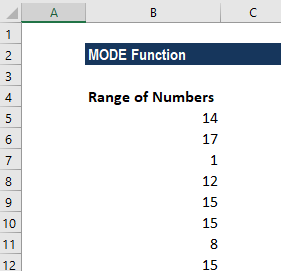The formula used was: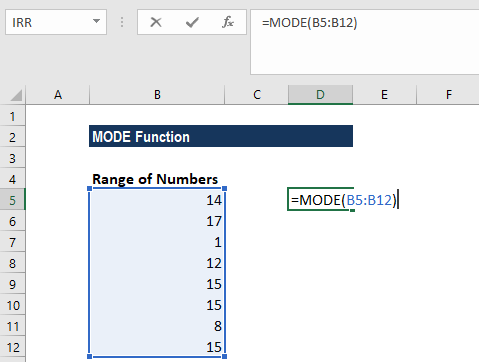We get the results below: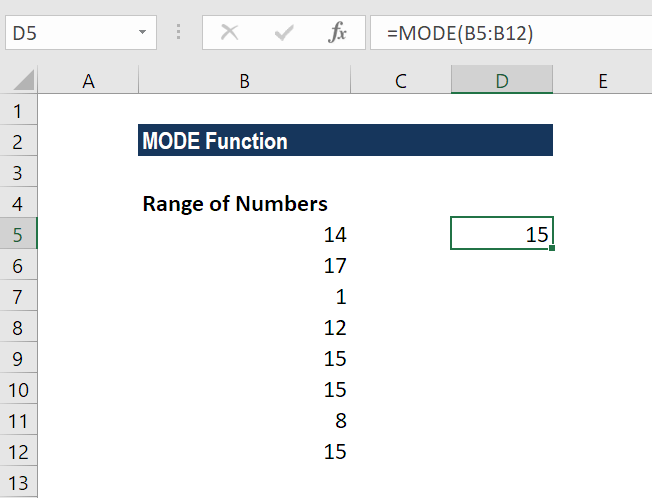#### Example 2

Suppose we are given the following data: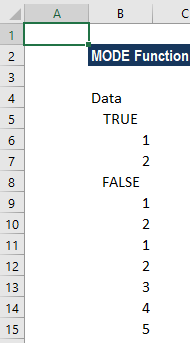In this case, there are two modes and logical values in the dataset.

The formula we used was: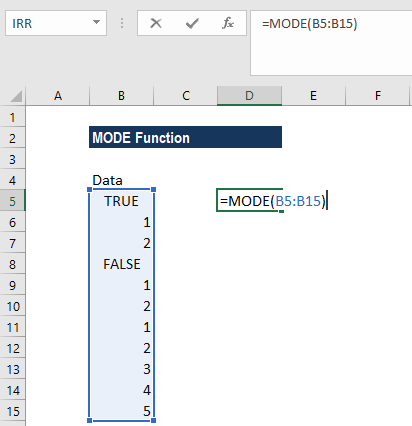While calculating MODE for the above range, the function ignored Logical values and gave the results below: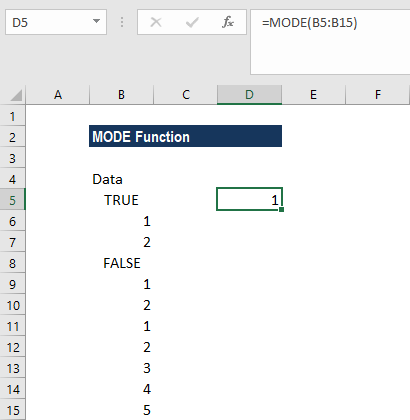In the above example, as the data given in column A show two statistical modes (1 and 2), the MODE function returns the lowest of the two values.

### Things to remember about the MODE Function

1. In Excel 2010, the MODE function was replaced by the MODE.SNGL function. Although it’s been replaced, current versions of Excel still provide the MODE function to allow compatibility with earlier versions. It is stored within the list of compatibility functions. However, the MODE function may not be available in future versions of Excel, so it is advised that you use the MODE.SNGL function whenever possible.
2. #NUM! error – Occurs if there are no duplicates and hence, there is no mode within the supplied values.
3. #VALUE! error – Occurs if a value that is supplied directly to the MODE is non-numeric and also is not part of an array. Non-numeric functions that are part of an array of values are ignored by the MODE function.

Thanks for reading CFI’s guide to important Excel functions! By taking the time to learn and master these functions, you’ll significantly speed up your financial modeling. To learn more, check out these additional CFI resources:

• Excel Functions for Finance
• Excel Shortcuts for PC and Mac
• What is Financial Modeling?
• Financial Modeling Guide

### Free Excel Tutorial

To master the art of Excel, check out CFI’s FREE Excel Crash Course, which teaches you how to become an Excel power user.  Learn the most important formulas, functions, and shortcuts to become confident in your financial analysis.

Launch CFI’s Free Excel Course now to take your career to the next level and move up the ladder!